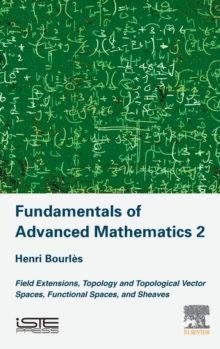# Fundamentals of Advanced Mathematics V2 : Field extensions, topology and topological vector spaces, functional spaces, and sheaves Hardback

#### Description

The three volumes of this series of books, of which this is the second, put forward the mathematical elements that make up the foundations of a number of contemporary scientific methods: modern theory on systems, physics and engineering. Whereas the first volume focused on the formal conditions for systems of linear equations (in particular of linear differential equations) to have solutions, this book presents the approaches to finding solutions to polynomial equations and to systems of linear differential equations with varying coefficients. Fundamentals of Advanced Mathematics, Volume 2: Field Extensions, Topology and Topological Vector Spaces, Functional Spaces, and Sheaves begins with the classical Galois theory and the theory of transcendental field extensions.

Next, the differential side of these theories is treated, including the differential Galois theory (Picard-Vessiot theory of systems of linear differential equations with time-varying coefficients) and differentially transcendental field extensions.

The treatment of analysis includes topology (using both filters and nets), topological vector spaces (using the notion of disked space, which simplifies the theory of duality), and the radon measure (assuming that the usual theory of measure and integration is known). In addition, the theory of sheaves is developed with application to the theory of distributions and the theory of hyperfunctions (assuming that the usual theory of functions of the complex variable is known).

This volume is the prerequisite to the study of linear systems with time-varying coefficients from the point-of-view of algebraic analysis and the algebraic theory of nonlinear systems.

#### Information

• Format: Hardback
• Pages: 360 pages
• Publisher: ISTE Press Ltd - Elsevier Inc
• Publication Date:
• Category: Mathematics
• ISBN: 9781785482496

£134.00

£115.75

on all orders

###### Pick up orders

from local bookshops